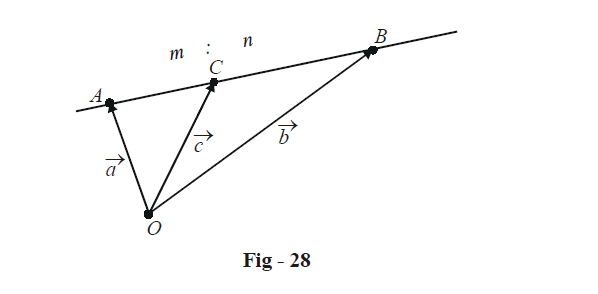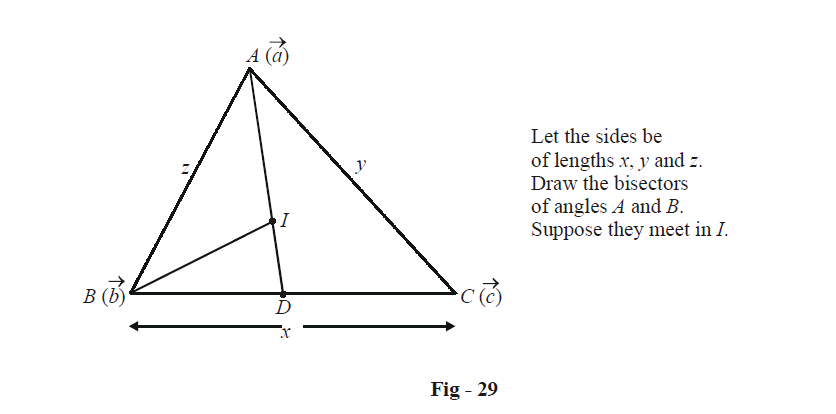# Examples On Basics Of Vectors Set-2

Go back to  'Vectors and 3-D Geometry'

Example – 9   SECTION FORMULA

Let $$A\left( {\vec a} \right)\,\,{\text{and}}\,B\left( {\vec b} \right)$$  be two fixed points. Find the position vectors of the points lying on the (extended) line AB which divide the segment internally and externally in the ratio m : n.

Solution: We consider internal division; the external division case follows analogously.

Let $$C\left( {\vec c} \right)$$ be the point which divides AB internally in the ratio m : n.We have,

\begin{align}&\quad\qquad\overrightarrow {AC} = \frac{m}{{m + n}}\,\,\,\overrightarrow {AB} \hfill \\\\&\Rightarrow \quad \left( {\overrightarrow {OC} - \overrightarrow {OA} } \right) = \frac{m}{{m + n}}\,\,\left( {\,\overrightarrow {OB} - \overrightarrow {OA} } \right) \hfill \\\\& \Rightarrow \quad \vec c - \vec a = \frac{m}{{m + n}}\,\,\left( {\vec b - \vec a} \right) \hfill \\\\& \Rightarrow \quad \boxed{\vec c = \frac{{m\vec b + n\vec a}}{{m + n}}\,} \hfill \\ \end{align}

Similarly, the point $$D(\vec d)$$ which divides AB externally in the ratio m : n is given by

$\vec d = \frac{{m\vec b - n\vec a}}{{m - n}}$

A particular case of internal division is the mid-point of $$A(\vec a)\,and\,B(\vec b)$$: the mid-point is \begin{align}\frac{{\vec a + \vec b}}{2},\end{align}

Example – 10

Show by vector methods that the angular bisectors of a triangle are concurrent and find the position of the point of concurrency in terms of the position vectors of the vertices.

Solution: Let the vertices of the triangle by $$A\left( {\vec a} \right),\,\,B\,(\vec b)\,{\text{and}}\,C(\vec c)$$. We use the geometrical fact that an angle bisector divides the opposite side in the ratio of the sides containing the angle.We thus have,

$\frac{{BD}}{{DC}} = \frac{c}{b}$

Thus, D is given by (the internal division formula):

$D \equiv \frac{{z\vec c + y\vec b}}{{z + y}}$

In $$\Delta$$ABD, since BI is the angle bisector, we have

$\frac{{DI}}{{IA}} = \frac{{BD}}{{BA}} = \frac{{\frac{z}{{z + y}}x}}{z} = \frac{x}{{z + y}}$

Thus, we now have the position vectors of A and D we know what ratio I divides AD in. I can now be easily determined using the internal division formula:

\begin{align}&\;\;I \equiv \frac{{x\vec a + \left( {x + y} \right)\left( {\frac{{z\vec c + y\vec b}}{{z + y}}} \right)}}{{x + z + y}} \hfill \\\\&\quad= \frac{{x\vec a + y\vec b + z\vec c}}{{x + y + z}}...{\text{ }}\left( 1 \right) \hfill \\ \end{align}

The symmetrical nature of this expression proves that the bisector of C will also pass through I. The angle bisectors will therefore be concurrent at I, called the incentre. The position vector of the incentre is given by (1).

Vectors
grade 11 | Questions Set 1
Vectors